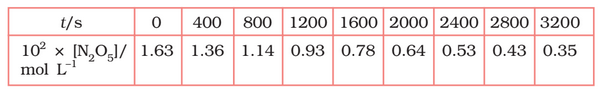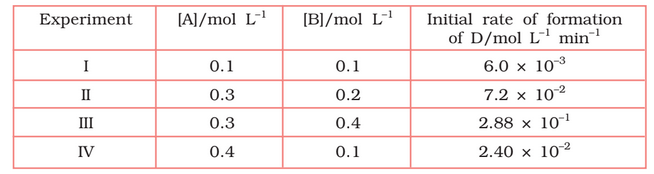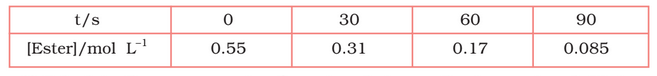## Filters

Sort by :
Clear All
Q

4.15 (1)    The experimental data for decomposition of

in gas phase at 318K are given below:Plot against t.

On increasing time, the concentration of  gradually decreasing exponentially.

4.14   The half-life for radioactive decay of is 5730 years. An archaeological artifact containing wood had only 80% of the 14C found in a living tree. Estimate the age of the sample.

Given , half-life of radioactive decay = 5730 years So,                  per year we know that, for first-order reaction,                  = 1845 years (approximately) Thus, the age of the sample is 1845 years

4.13 (3)   Calculate the half-life of a first order reaction from their rate constants given  below:

The half-life for the first-order reaction is                                                                               = 0.693/4                                                                             =  0.173 year (approximately)

4.13 (2)   Calculate the half-life of a first order reaction from their rate constants given below:

the half-life for the first-order reaction is expressed as ;           =  0.693/2           = 0.35 min (approx)

4.13 (1)   Calculate the half-life of a first order reaction from their rate constants given below:

We know that, half-life () for first-order reaction =                                                             =

4.12   The reaction between A and B is first order with respect to A and zero order
with respect to B. Fill in the blanks in the following table:The given reaction is first order wrt A and zero order in wrt B. So, the rate of reaction can be expressed as;   Rate = k[A] from exp 1, k = 0.2 per min. from experiment 2nd, [A] =  from experiment 3rd,             from the experiment 4th, from here [A] = 0.1 mol/L

4.11   The following results have been obtained during the kinetic studies of the reaction:
2A + B  C + DDetermine the rate law and the rate constant for the reaction.

Let assume the rate of reaction wrt A is  and wrt B is  . So, the rate of reaction is expressed as- Rate =  According to given data,  these are the equation 1, 2, 3 and 4 respectively Now,  divide the equation(iv) by (i) we get, from here we calculate that  Again, divide equation (iii) by (ii) from here we can calculate the value of y is 2 Thus, the rate law is now,   So,         ...

4.10   In a reaction between A and B, the initial rate of reaction (r0) was measure for different initial concentrations of A and B as given below:What is the order of the reaction with respect to A and B?

we know that  rate law () =  As per data   these are the equation 1, 2 and 3 respectively Now, divide eq.1 by equation2, we get from here we calculate that y = 0 Again, divide eq. 2 by Eq. 3, we get Since y =0 also substitute the value of y  So,   =  =  taking log both side we get,     = 1.496     = approx 1.5 Hence the order of reaction w.r.t A is 1.5 and w.r.t B is 0(zero)

4.8 In pseudo first order hydrolysis of ester in water, the following results were obtained:(i) Calculate the average rate of reaction between the time interval 30 to 60 seconds.

The average rate of reaction between the time 30 s to 60 s is expressed as-

4.9     A reaction is first order in A and second order in B.

(iii) How is the rate affected when the concentrations of both A and B are doubled?

If the concentration of [A] and[B] is increased by 2 times, then                 Therefore, the rate of reaction will increase 8 times.

.
4.9    A reaction is first order in A and second order in B.

(ii) How is the rate affected on increasing the concentration of B three times?

If the concentration of [B] is increased by 3 times, then                 Therefore, the rate of reaction will increase 9 times.

4.9     A reaction is first order in A and second order in B.

(i)Write the differential rate equation.

the reaction is first order in A and second order in B. it means the power of A is one and power of B is 2 The differential rate equation will be-

4.7     What is the effect of temperature on the rate constant of a reaction? How can this effect of temperature on rate constant be represented quantitatively?

The rate constant is nearly double when there is a 10-degree rise in temperature in a chemical reaction. effect of temperature on rate constant be represented quantitatively by Arrhenius equation,  where k is rate constant                                                                                                            A is Arrhenius factor           R is gas  constant                 ...

4.6    A reaction is second order with respect to a reactant. How is the rate of reaction affected if the concentration of the reactant is  reduced to half ?

Let assume the concentration of reactant be x So, rate of reaction, R =  Now, if the concentration of reactant is doubled then  . So the rate of reaction would  be   Hence we can say that the rate of reaction reduced to 1/4 times.

4.6     A reaction is second order with respect to a reactant. How is the rate of reaction affected if the concentration of the reactant is doubled

Let assume the concentration of reactant be x So, rate of reaction,   Now, if the concentration of reactant is doubled then  . So the rate of reaction would  be   Hence we can say that the rate of reaction increased by 4 times.

4.5     Mention the factors that affect the rate of a chemical reaction.

The following factors that affect the rate of reaction- the concentration of  reactants  temperature, and presence of catalyst

4.4  The decomposition of dimethyl ether leads to the formation of ,  and   and the reaction rate is given by
The rate of reaction is followed by increase in pressure in a closed vessel, so the rate can also be expressed in terms of the partial pressure of dimethyl ether, i.e.,

If the pressure is measured in bar and time in minutes, then what are the units of rate and rate constants?

Given that  So, the unit of rate is bar/min.() And thus the unit of k = unit of rate

4.3     The decomposition of  on platinum surface is zero order reaction. What are the rates of production of  and if ?

The decomposition of  on the platinum surface reaction  therefore,    Rate =  For zero order reaction rate = k therefore,  So    and the rate of production of dihydrogen  = 3(2.5)                                                                             = 7.5

4.2     For the reaction:

the rate = with . Calculate the initial rate of the reaction when. Calculate the rate of reaction after  is reduced to .

The initial rate of reaction =   substitute the given values of [A], [B] and k, rate  =          =8 When [A] is reduced from 0.1 mol/L to 0.06 mol/L  So, conc. of A reacted = 0.1-0.06 = 0.04 mol/L and conc. of B reacted = 1/2(0.04) = 0.02mol/L conc. of B left = (0.2-0.02) = 0.18 mol/L     Now,  the rate of the reaction is (R) =                                                                  ...

4.1  From the rate expression for the following reactions, determine their order of reaction and the dimensions of the rate constants.

so the order of the reaction is 1 and the dimension of k =
Exams
Articles
Questions